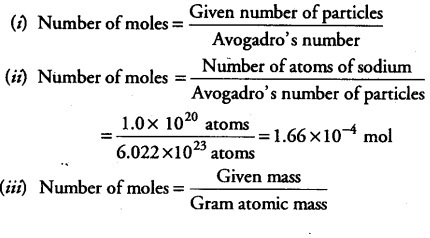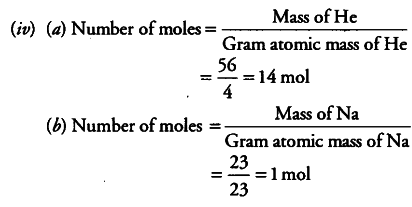# How do we express number of particles in - terms of moles?

(i) How do we express number of particles in - terms of moles?
(ii) Calculate the number of moles of sodium in a sample containing 1020 atoms of sodium.
(iii) How do we express mass of a substance in terms of moles?
(iv) Calculate the number of moles in
(a) 56 g of He (b) 23 g of Na